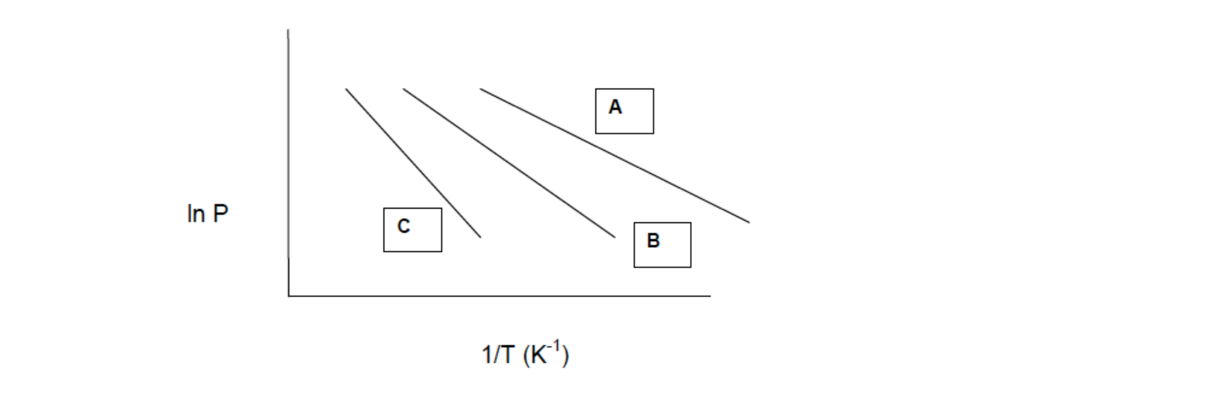# Problem: Which compound has the lowest boiling point?

###### FREE Expert Solution
92% (402 ratings)
###### Problem Details

Which compound has the lowest boiling point?Frequently Asked Questions

What scientific concept do you need to know in order to solve this problem?

Our tutors have indicated that to solve this problem you will need to apply the Clausius-Clapeyron Equation concept. You can view video lessons to learn Clausius-Clapeyron Equation. Or if you need more Clausius-Clapeyron Equation practice, you can also practice Clausius-Clapeyron Equation practice problems.

What is the difficulty of this problem?

Our tutors rated the difficulty ofWhich compound has the lowest boiling point? ...as low difficulty.

How long does this problem take to solve?

Our expert Chemistry tutor, Jules took 1 minute and 13 seconds to solve this problem. You can follow their steps in the video explanation above.

What professor is this problem relevant for?

Based on our data, we think this problem is relevant for Professor Van Dorn's class at ARIZONA.